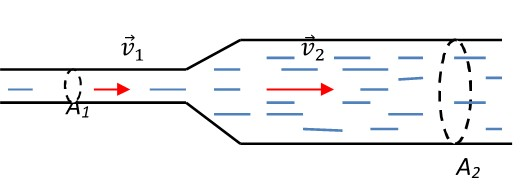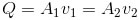﻿ All The formulas related to Mechanics Of Fluids

# List of formulas related to Mechanics Of Fluid

#### Density

m: mass of a volume V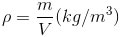#### Pressure

F: force applied on area of A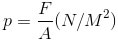#### Pressure Of Liquids

d: specific gravity(N/m3), po: air pressure at the free surface (N/m2), p: pressure created by the weight of liquid above, pB: total pressure at the depth h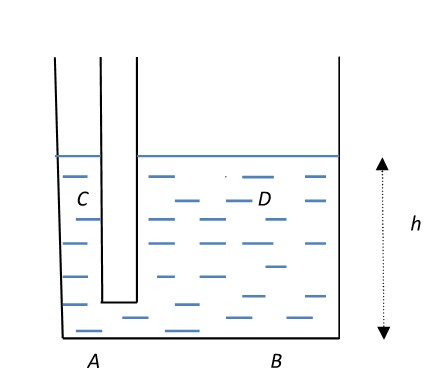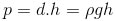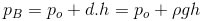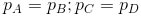#### Pascal's Principle

PS: pressure on the surface

>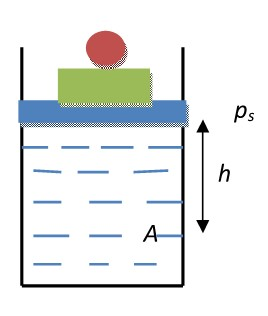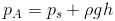#### Application

A1, A2: area of small and large cylinders; F1, F2: applied and upward forces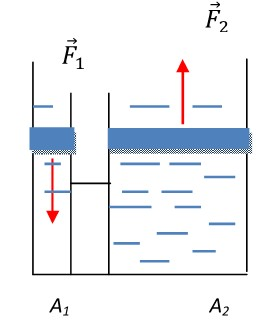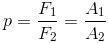#### Archimede's Principle

d: specific gravity of liquid (N/m3), V: volume of object, Fb: buoyant force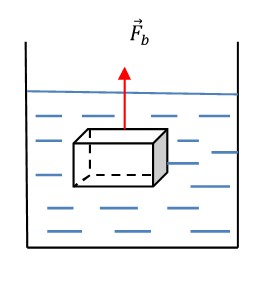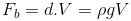#### Flow Rate

A: cross sectional area (m2), v: flow velocity (m/s), Q: flow rate (m3/s)